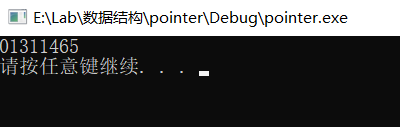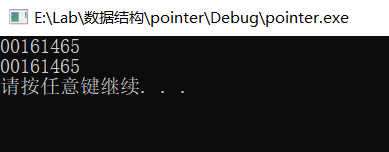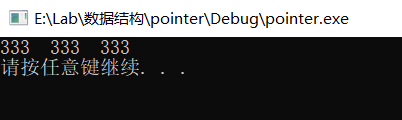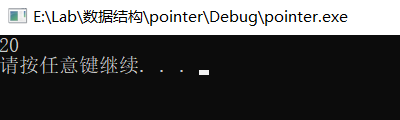# 指针与函数C语言指针 同时被 2 个专栏收录10 篇文章 1 订阅

## 1.指针可以调用函数

int sum(int a, int b)
{
return a + b;
}

int main()
{
printf("%p\n", sum);

system("pause");
return 0;
}## 2.函数指针变量定义

int sum(int a, int b) {}

int (*pSum)(int a, int b);  //函数指针变量，参数名可以省略

pSum = sum;  //给指针赋地址值
printf("%p\n", sum);

int(*pSum)(int, int);
pSum = sum;

printf("%p\n", pSum);## 3.函数指针类型定义

typedef int(*PSUM)(int, int);
PSUM pSum2 = sum;
PSUM pSum3 = sum;

## 4.函数指针的使用

int(*pSum)(int, int);
pSum = sum;

typedef int(*PSUM)(int, int);
PSUM pSum2 = sum;

cout << sum(111, 222) << "  " << pSum(111, 222) << "  " << pSum2(111, 222) << endl;## 5.多级复杂函数指针

#include <iostream>
using namespace std;

//定义一个函数，参数为int型，返回输入的倍数，也是一个int型
int fun0(int a)
{
return a + a;
}

//定义一个函数指针类型，参数为一个int型，返回为int
typedef int(*PFUN)(int);

//该函数返回一个函数指针，参数为int型
PFUN fun(int a)
{
return fun0;
}

int main()
{
int(*(*p)(int))(int);
p = fun;
cout << p(111)(10) << endl;

system("pause");
return 0;
}1）解释一下p(111)(10)的含义，首先p(111)调用函数fun，该函数返回函数fun0的地址，在函数的地址后面加上(10)，表示输入参数10，最后返回20

2）解释一下为什么在定义函数指针类型的时候要返回值int，即typedef int (*PFUN)(int);里面的第一个int，如果使用float行不呢，答案肯定是不行的，这里的int依据的是定义函数指针p那里最左边的int，即int (* (* p)(int) )(int);里面的第一个int，要求返回int。如果我们改成float (* (* p)(int) )(int);那么相应的函数指针类型那里也要改成float，即typedef float (*PFUN)(int);最后在定义函数fun0那里，也要改成返回float型

04-18
09-041万+03-23431
03-303万+
11-2410万+
05-2417万+
03-0965
11-05137
11-216万+
04-2149
11-182282
04-23210
02-286990
04-091万+
07-25336
03-18617
08-021264点击重新获取扫码支付余额充值